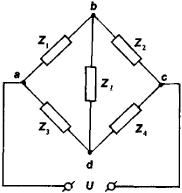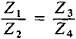# Bridge Circuit

Also found in: Dictionary, Thesaurus, Medical, Legal, Financial, Wikipedia.

## Bridge circuit

A circuit composed of a source and four impedances that is used in the measurement of a wide range of physical quantities. The bridge circuit is useful in measuring impedances (resistors, capacitors, and inductors) and in converting signals from transducers to related voltage or current signals. See Capacitor, Inductor, Resistor

The bridge impedances Z1, Z2, Z3, Z4, shown in the illustration may be single impedances (resistor, capacitor, or inductor), combinations of impedances, or a transducer with varying impedance. For example, strain gages are resistive transducers whose resistance changes when they are deformed.

Bridge circuits are often used with transducers to convert physical quantities (temperature, displacement, pressure) to electrical quantities (voltage and current). High-accuracy voltmeters and ammeters are relatively inexpensive, and the voltage form of a signal is usually most convenient for information display, control decisions, and data storage. Another important advantage of the bridge circuit is that it provides greater measurement sensitivity than the transducer.

The bridge circuit is balanced when the output read by the meter is zero. In this condition the voltages on both sides of the meter are identical. The bridge is used in two forms. The null adjustment method requires adjustment of a calibrated impedance to balance it. In this case the meter is usually a highly sensitive current-measuring galvanometer. The null adjustment method is often used to measure impedances, with the output read from a dial attached to the adjustable impedance. The deflection method requires on accurate meter in the bridge to measure the deviation from the balance condition. The deviation is proportional to the quantity being measured.

There are many special forms of the bridge circuit. When all of the impedances are resistive, it is commonly called a Wheatstone bridge. Other common forms use a current source in place of the voltage source, a sinusoidal source in place of a constant (dc) source, or branch impedances which are specific combinations of single passive impedances. The bridge circuit is also used in a variety of electrical applications varying from oscillators to instrumentation amplifier circuits for extremely accurate measurements. See Wheatstone bridge

McGraw-Hill Concise Encyclopedia of Physics. © 2002 by The McGraw-Hill Companies, Inc.
The following article is from The Great Soviet Encyclopedia (1979). It might be outdated or ideologically biased.

## Bridge Circuit

(or electric bridge), an electric four-terminal network. One pair of its terminals (poles) is connected to a power supply, and the other pair, to a load. The classical bridge circuit consists of four resistances connected in series and forming a quadrilateral (see Figure 1), points a, b, c, and d of which are called vertexes. The arm containing the power supply U is called the supply diagonal, and the arm containing the load resistance Z1 is called the load diagonal or indicator diagonal. The resistances Z1,, Z2, Z3 and Z4, each connected between two adjacent vertexes, are called the arms of the bridge circuit. The diagonals of a bridge circuit connect, or bridge, two opposite vertexes (for this reason the load diagonal was formerly called the bridge). The circuit shown in Figure 1 is known in the literature as a Wheatstone or four-arm bridge.Figure 1. Diagram of a Wheatstone (four-arm) bridge

The potential difference between points b and d—and consequently the current in the load diagonal—will be equal to zero for any value of the electromotive force (emf) of the power supply if the resistances of the bridge arms satisfy the equationThis equation is called the equilibrium condition for a Wheatstone bridge. In DC bridge circuits balance can be achieved by adjusting the resistance of one of the arms. In AC bridge circuits the equilibrium condition involves complex quantities; the condition can be broken down into two equations, each of which involves real numbers (the parameters of the arms, such as resistance, inductance, capacitance, and frequency). Therefore, the balancing of an AC bridge circuit usually requires the adjustment of at least two parameters of the bridge.

A distinction is made between bridge circuits in which the balance depends on the frequency of the supply voltage and circuits that remain balanced at any power-supply frequency. The equilibrium conditions for frequency-dependent bridge circuits contain the frequency of the supply voltage as one of the variables. Balanced bridge circuits are used predominantly for measuring electric resistances, capacitances, and inductances. In combination with primary parametric converters they are also widely used as means of measuring nonelectrical quantities, such as temperature, deformation, and acceleration.

Bridge circuits operating in the unbalanced mode are often used as converters; their output is current or voltage in the load diagonal. AC bridge circuits may also be operated in an unbalanced or quasi-balanced mode. An important type of bridge circuit is the three-terminal network, which is used to protect circuits from interference and crosstalk, particularly at high frequencies.

Bridge circuits have become widespread in various electrotechnical and radio-engineering devices. An example of such applications is the parametric voltage regulator, which is a Wheatstone bridge with a nonlinear resistance in one of the arms. In such a circuit, the voltage in the load diagonal is not highly dependent on fluctuations in the supply voltage. This type of regulator operates equally well on direct and alternating current. Another example is a current rectifier, which is constructed as a bridge circuit and used to convert alternating current to direct current. Bridge circuits are also used in metrology, automation, remote control, computer engineering, and communications engineering.

### REFERENCES

Karandeev, K. B. Mostovye metody izmerenii Kiev, 1953.
Beletskii, A. F. Osnovy teorii lineinykh elektricheskikh tsepei. Moscow, 1967.
Smolov, V. B. , and E. L. Kantor. Mostovye vychislitel’nye ustroistva. Leningrad, 1971. Osnovy elektroizmeritel’noi tekhniki. Moscow, 1972.

V. N. MALINOVSKII

## bridge circuit

[′brij ‚sər·kət]
(electricity)
An electrical network consisting basically of four impedances connected in series to form a rectangle, with one pair of diagonally opposite corners connected to an input device and the other pair to an output device.
McGraw-Hill Dictionary of Scientific & Technical Terms, 6E, Copyright © 2003 by The McGraw-Hill Companies, Inc.

## Bridge circuit

A circuit composed of a source and four impedances that is used in the measurement of a wide range of physical quantities. The bridge circuit is useful in measuring impedances (resistors, capacitors, and inductors) and in converting signals from transducers to related voltage or current signals. See Capacitor, Inductor, Transducer

The bridge impedances Z1, Z2, Z3, Z4, shown in the illustration may be single impedances (resistor, capacitor, or inductor), combinations of impedances, or a transducer with varying impedance. For example, strain gages are resistive transducers whose resistance changes when they are deformed.

Bridge circuits are often used with transducers to convert physical quantities (temperature, displacement, pressure) to electrical quantities (voltage and current). High&hyphen;accuracy voltmeters and ammeters are relatively inexpensive, and the voltage form of a signal is usually most convenient for information display, control decisions, and data storage. Another important advantage of the bridge circuit is that it provides greater measurement sensitivity than the transducer.

The bridge circuit is balanced when the output read by the meter is zero. In this condition the voltages on both sides of the meter are identical. The bridge is used in two forms. The null adjustment method requires adjustment of a calibrated impedance to balance it. In this case the meter is usually a highly sensitive current-measuring galvanometer. The null adjustment method is often used to measure impedances, with the output read from a dial attached to the adjustable impedance. The deflection method requires on accurate meter in the bridge to measure the deviation from the balance condition. The deviation is proportional to the quantity being measured.

There are many special forms of the bridge circuit. When all of the impedances are resistive, it is commonly called a Wheatstone bridge. Other common forms use a current source in place of the voltage source, a sinusoidal source in place of a constant (dc) source, or branch impedances which are specific combinations of single passive impedances. The bridge circuit is also used in a variety of electrical applications varying from oscillators to instrumentation amplifier circuits for extremely accurate measurements. See Instrumentation amplifier, Oscillator

McGraw-Hill Concise Encyclopedia of Engineering. © 2002 by The McGraw-Hill Companies, Inc.
References in periodicals archive ?
Magnetic coupling measurements between coils were repeated by placing 1 mm of pork skin, 1 mm of fat, and 2 mm of pork muscle over the Maxwell-Wien bridge circuit. The distance between coils was swept this time from 20 mm to 5 mm.
However, during experiments aimed to confirm the correctness of measurement of the two mentioned non-electrical quantities by the two-current-source bridge circuit, certain difficulties occurred.
The gages are wired in a Wheatstone bridge circuit, including bridge completion resistors when needed, so the pressure gage is electrically equivalent to a load cell.
Two-phase circuits require a blocking capability of three times the supply voltage to handle inductive transients during turn-off, whereas lower bridge circuit ratings may be permissible (for example, twice the supply).
In order to control the compensation unit a synchronization with the main, full bridge circuit is required.
(If PEC is included then the additional losses would need to be included for the bridge circuit.)
These bridge circuits are preferred in dynamic measurements where the frequency band is wide and, even with a big change in the sensor resistance, the amplifier output voltage is not in saturation.
Part 1 explains how to build a monitoring systems from simple to complex instrumentation, covering basic circuits and bridge circuits of sensors and transducers, signal conditioning, data acquisition systems, single-board computers and microcontrollers, wireless technologies and telemetry, wireless sensor networks, and databases and web access.

Site: Follow: Share:
Open / Close